# Java Program to Find all Roots of a Quadratic Equation

In this tutorial you will learn about the Java Program to Find all Roots of a Quadratic Equation and its application with practical example.

In this tutorial, we will learn to create a Java program that will Find all Roots of a Quadratic Equation using Java programming.

## Prerequisites

Before starting with this tutorial, we assume that you are the best aware of the following Java programming topics:

• Operators in java programming.
• Basic input/output in java programming.
• Basic in java programming.
• Inbuilt library functions in java programming.

## What is a Quadratic Equation?

In particular, it is a second-degree polynomial equation, since it has the greatest power is two.In algebra said a quadratic polynomial, a polynomial of degree 2.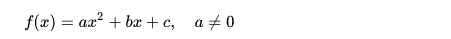## Java Program to Find All Roots of a Quadratic Equation

In this program, we will Find All Roots of a Quadratic Equation. First of all we declare and initialize the required variables. Next, we would prompt the user to input the values of a,b, and c then with the help of sqrt() function we will calculate the first and second root or equations.

## Output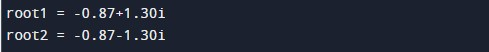In the above program, we have first declared and initialized a set variables required in the program.

• a,b,c = for holding coordinates
• root1= shows first root.
• root2= shows the second root.

In this program first of we prompted user to input values of a,b and c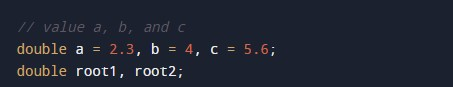after getting the values of a,b and c and with the help of inbuilt function sqrt() and mathematical formula we know to calculate all Roots of a Quadratic Equation.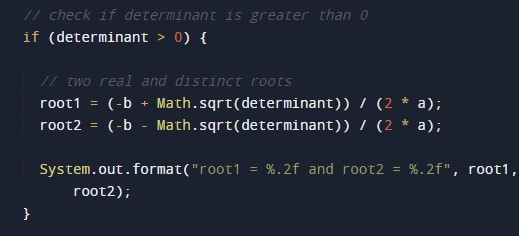whose value define in math.h header file we going to find the Second Order Quadratic Equation.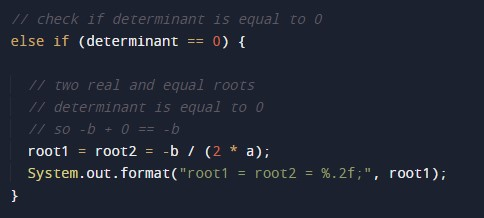As we know the formula of Quadratic Equation. we will use formula to get first and second root of given coordinates using Java program.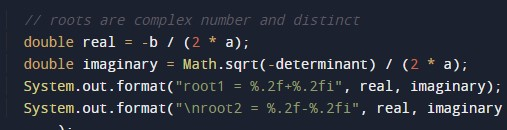In this tutorial we have learn about the Java Program to Find all Roots of a Quadratic Equation and its application with practical example. I hope you will like this tutorial.